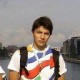# Get/Set local variables of JavaScript function from outside & Dynamic code insertion in JS function

I am working on a JavaScript framework that needs to get list of local function variables and set values of some of them. Unlike Java, JavaScript does not have runtime introspection
support. In Java you can do this using Java Reflection APIs, but I couldn’t think of any way to do this in JavaScript. So I searched on the web and came across two threads of discussions on StackOverflow –

There are some interesting solutions discussed in the above threads. The first one discusses solutions for setting local variable values. The second one interested me because I found the technique useful for injecting code (that will set local variable value) in a function.

However they do not directly provide solution for getting local variables in a JS function from outside it. But I was able to come up with a solution for this. The scenario for which I have posted the code below is this – a function, let’s call it ‘test’, declares a few local variables, prints value of a variable before it calls a framework function (which gets local variables declared in the ‘test’ function and changes value of a variable) and then prints the value of the same variable (after it has been changed by the framework function). Here is the complete solution/code –

```<script language="JavaScript">
//Instrument local functions for the framework
instrumentFunctions();

/**
* Test function.
* We will get all local variables in this function and set value of a local variable
*/
function test()
{
//Declare some local variables
var i = 100;
var  j = 10, k = 20;

//Print value of i before it is changed by our framework
console.log(" i = " + i);
document.writeln("Before framework call, i = " + i + "<br>");

//Call a framework specific function.
//For this example, we will change value of i in the framework function
if (typeof __ctx != 'undefined')
someFrameworkFunction(__ctx);

//Print value of i after calling framework function
console.log(" i = " + i);
document.writeln("After framework call, i = " + i + "<br>");
}

/**
* Some framework function that is expected to work with local variables declared in the
* function from which it is called.
*
* @param {Object} ctx - context object that has reference to caller and code injected by instrumentFunc function
*/
function someFrameworkFunction(ctx)
{
//Assume this is some framework function that needs to access
//and set local variables of calling function.

//Get list of local variables in a function from which this function is called
var localVars = ctx.getLocalVars();
//Print local variables
document.writeln("Local variables in " + ctx.caller.name + " : " + localVars.toString() + "<br>");

//Change value of local variable i
ctx.set("i", 300);
}

/**
* Calls instrumentFunction method for functions that need to be instrumented
*/
function instrumentFunctions()
{
//Instrument all functions that you want to be accessed by the framework
instrumentFunc(test);
}

/**
* Inserts code in the given function to set local variable and get list of local variables
*
* @param {Function} func - Function in which to insert framework specific code
*/
function instrumentFunc(func)
{
if (typeof func != 'function')
return;

var oldCode = func.toString();

//We want to insert code in the function just after opening '{'
var index = oldCode.indexOf("{");

//Split code, part before and after opening '{'
var oldCodePart1 = oldCode.slice(0,index+1);
var oldCodePart2 = oldCode.slice(index+1);

//We will create a context object and create keys to set and get local variables.
var codeToInsert = "var __ctx = {}; __ctx.caller=arguments.callee;__ctx.variables={};__ctx.set = __setVar;";

//setVar function declaration
codeToInsert += "function __setVar(name, value){try{eval(name);} catch (err){return false;}eval(name + \" = \" + value);return true;};";
codeToInsert += "__ctx.getLocalVars = __getLocalVars;"

//setLocalVars function declaration
codeToInsert += "function __getLocalVars(){return getLocalVarNames(__ctx.caller.toString());};"

//New function code
var newCode = oldCodePart1 + codeToInsert + oldCodePart2;

//Create new function
var newFunc = eval("newCode");

//Replace old function
eval ("window." + func.name + " = " + newFunc);
}

/**
* Gets local variables, declared with var keyword in the given code
*
* @param {String} code - JS code
*
* @returns Array of local variable names
*/
function getLocalVarNames (code)
{
//Find variables declared as var, i.e. local variables in the given code
var regex =/\bvar\b\s*(?:\s*(\w+)\s*(?:=[^,;]+)?)(?:\s*,\s*(\w+)\s*(?:=[^,;]+)?)*\s*;/g;
var matches, result = [];
while (matches = regex.exec(code))
{
for (var i = 1; i < matches.length; i++) {
if (matches[i] != undefined) {
//filter framework specific variables here
if (matches[i] != "__ctx")
result.push(matches[i]);
}
}
}
return result;
}

//Call test function. This function is already instrumented in instrumentFunctions
test();

</script>```

-Ram Kulkarni

## 3 Replies to “Get/Set local variables of JavaScript function from outside & Dynamic code insertion in JS function”

1. Pingback: Cathy
2.Pippo says:

It seems to work in FF24, but not in IE10.

3.Alex says:

Really nice solution !